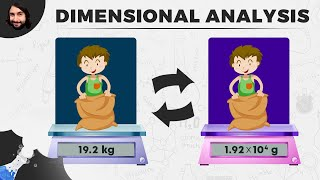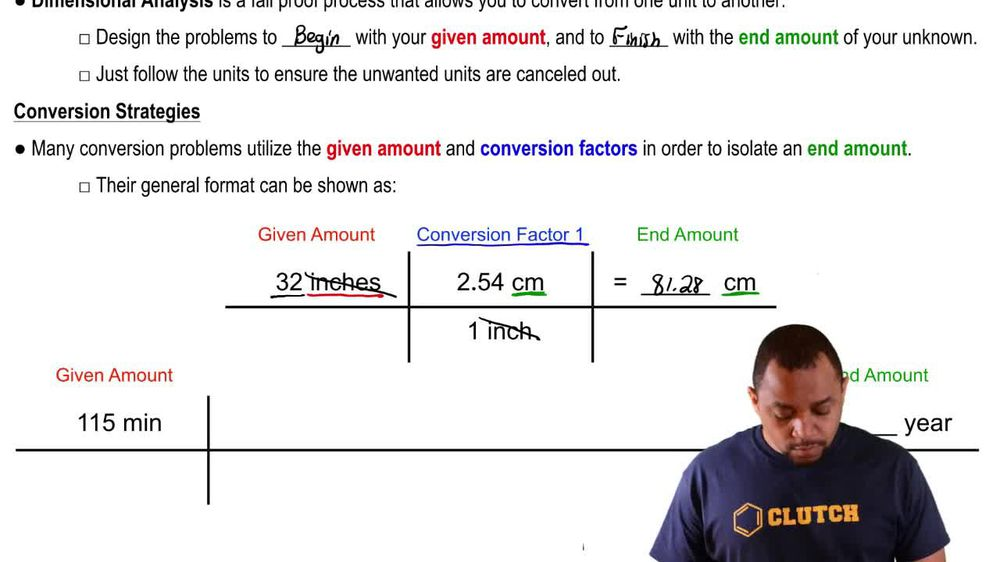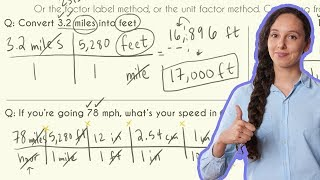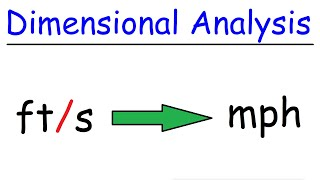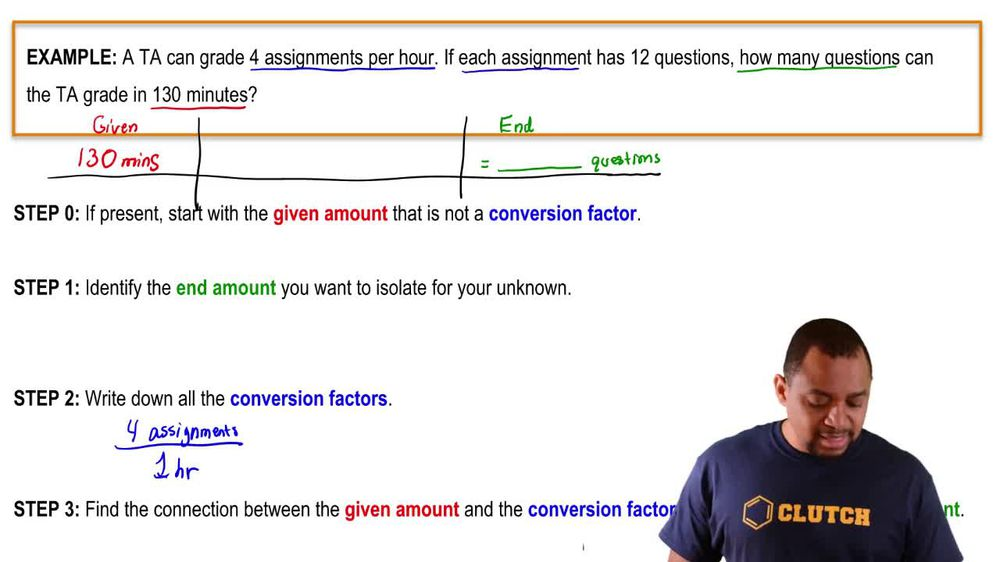Start typing, then use the up and down arrows to select an option from the list.
1. 1. Intro to General Chemistry2. Dimensional Analysis
Problem

# The distance from Earth to the Moon is approximately 240,000 mi. (d) Earth travels around the Sun at an average speed of 29.783 km>s. Convert this speed to miles per hour.

Relevant Solution1m
Play a video:
Hey everyone. So here it says, the Earth revolves at a speed of 30.012 km/s around the sun. Now, if the earth is approximately 256,000 miles away from the sun, how fast does it revolve in mph? Alright, so this is a common type of idea where they're giving us a bunch of information and sometimes some of it's there just to distract us the fact that they're telling us how far the Earth is from the sun has nothing to do with this question. This doesn't matter, this is how fast it's revolving in kilometers per second. All they want to know is how is it revolving in terms of MPH? So we're only concerned with this number and all we have to do is convert the units Again at 256,000 miles away from the sun is just extra information we can ignore. So we have 30.12 kilometers per hour, so that's per one per one second for one second and we're gonna stay here, let's change our seconds into hours. So we want seconds to go away. So it goes here on the top. So we have 60 seconds for one minute. Then we can say here that it's 16 minutes per one hour and we just converted our seconds into hours that we need. Next, we have to convert km two miles. We want to get rid of km. So we put kilometers here on the bottom and the conversion factor is one km, this .6-1371 mi. So at the end we're gonna have our units in MPH. When we plug that in, we get 67,134.91 mph or so. Here are closest answer to that would be Option B. So option B is our final answer.